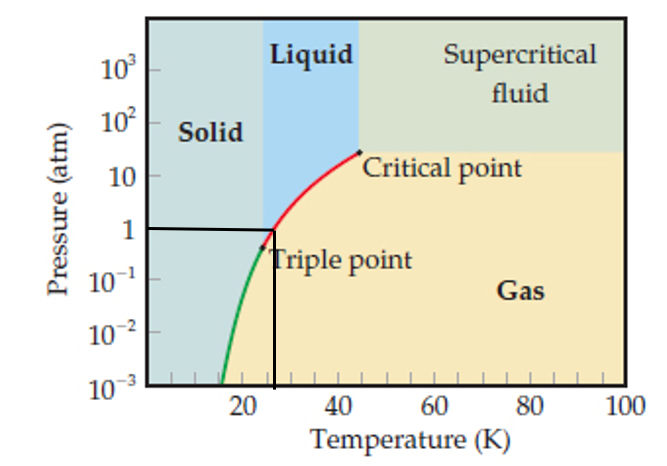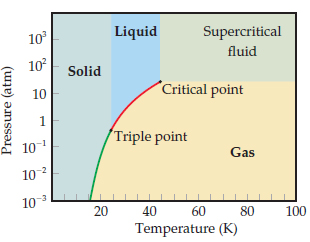# Problem: Use the phase diagram of neon  to answer the following questions.What is the approximate value of the normal boiling point?

###### FREE Expert Solution

boiling point at 1 atm97% (286 ratings)###### Problem Details

Use the phase diagram of neonto answer the following questions.

What is the approximate value of the normal boiling point?

Frequently Asked Questions

What scientific concept do you need to know in order to solve this problem?

Our tutors have indicated that to solve this problem you will need to apply the Phase Diagram concept. You can view video lessons to learn Phase Diagram. Or if you need more Phase Diagram practice, you can also practice Phase Diagram practice problems.

What professor is this problem relevant for?

Based on our data, we think this problem is relevant for Professor Fakhreddine's class at TEXAS.